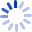# Mathematical Physics

"Mathematical Physics" is an introduction to such basic mathematical structures as groups, vector spaces, topological spaces, measure spaces, and Hilbert space. Geroch uses category theory to emphasize both the interrelationships among different structures and the unity of mathematics. Perhaps the most valuable feature of the book is the illuminating intuitive discussion of the "whys" of proofs and of axioms and definitions. This book, based on Geroch's University of Chicago course, will be especially helpful to those ...

##### Cash for Textbooks